# Table 2: Explicit description of the moves to solve the N=3 MToH

 Page 5/20 Date 23.04.2018 Size 2.78 Mb. #45758

Table 2: Explicit description of the moves to solve the N=3 MToH puzzle. The total number of moves is 11, which does NOT exactly coincide with the "base 3 rule" (Equation 5).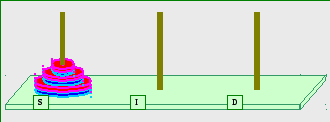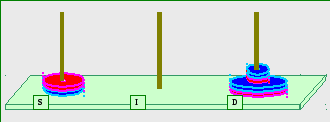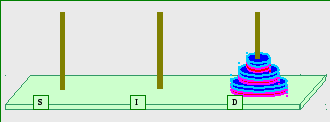Figure 5: The start-setting (top), an intermediate setting (center) and the end-state (bottom) for the N=3 MToH puzzle. The number of moves to progress from the start-setting to the intermediate state described by the center figure is 8 (read the text for details). The number of moves to progress from the center-described state to the end-state described by the bottom figure is 3. Thus, the number of moves required to solve the puzzle is S(3) = 11. The S(3) number (11) breaks the perfect base 3 rule (Equation 5). We therefore need to probe into the puzzle further in order to decipher the mystery of this newly observed irregularity (and come up with a modified rule).

Listed in table 2 are the moves required to solve the N=3 MToH puzzle.

As shown in Table 2 and as demonstrated by Figure 5 –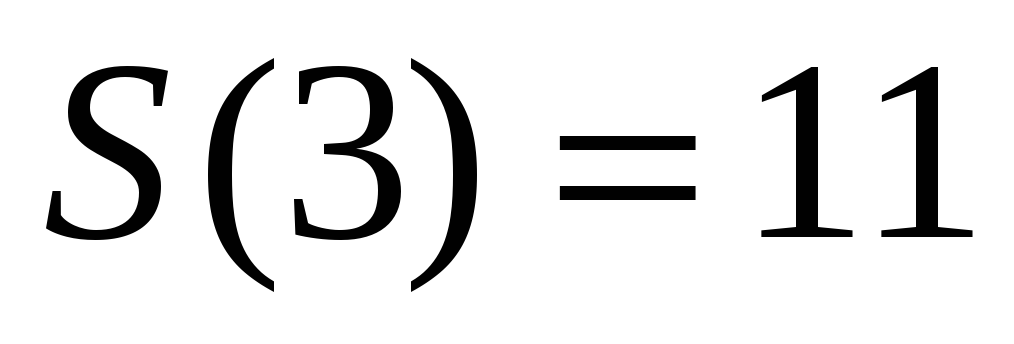. (6)
The resulting total number of moves violates the "base 3 rule" (should have been 13, refer to Equation 5). The states of the puzzle before step 1 (puzzle-start state), after step 4 and after step 7 (puzzle-end state) are shown by Figure 5 – top, center, bottom respectively.

In order to decipher the mystery (of the newly observed irregularity) and to progress with the analysis of the MToH puzzle, let's define a new magnetic tower, refer to it as the "Colored Magnetic Tower of Hanoi" and study its properties.

1. The Colored Magnetic Tower of Hanoi – the "100" solution

Studying the N=3 MToH puzzle, I realized that what breaks the base 3 rule is the possibility of the smallest disk to move to a free post (step 5 in Table 2). By "free" I mean a post that is not "magnetized" or not "color coded". A Neutral Post that can accept any-color disk. To suppress this freedom, let's permanently color-code each post, call the restricted tower the Colored Magnetic Tower of Hanoi (CMToH) and see what happens.

1. Definition of "Colored"

An MToH is "Colored" if (without loss of generality) its posts are pre-colored (or "permanently colored")

Either as

1. Red-Blue-Blue

Or as

2. Red-Red-Blue.
Let's designate this (permanently) Colored MToH as CMToH.

The two versions of the newly defined CMToH puzzle are shown by Figure 6. The moves to solve the CMToH puzzle with N=2, for each of its versions, are explicitly detailed by Table 3. Note that the only difference between the versions is the "timing" of the move of the big disk (after one move of the small disk in the first version and after two moves of the small disk in the second version).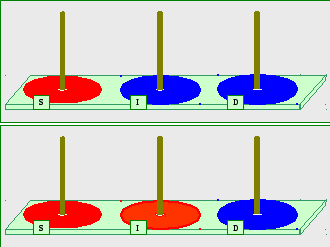Figure 6: The two versions of the (permanently) Colored Magnetic Tower of Hanoi. As shown in the text, the two definitions are equivalent in terms of number of moves. Given a Colored Magnetic Tower of Hanoi, the number of moves of disk k are P(k) = 3(k-1) and the total number of moves is S(N) = (3N – 1)/2. Thus, the freshly defined Colored Magnetic Tower of Hanoi strictly spans base 3.

 Step number Disks From To # of moves Comments 1. Red-Blue-Blue 1 2 S (Red) I (Blue) 1 2 1 S (Red) D (Blue) 1 3 2 I (Blue) D (Blue) 2 Through Red S 2. Red-Blue-Red 1 2 S (Red) I (Red) 2 Through Blue D 2 1 S (Red) D (Blue) 1 3 2 I (Red) D (Blue) 1